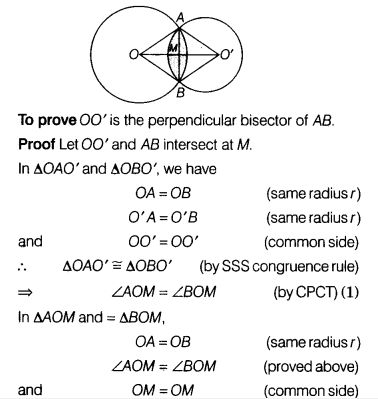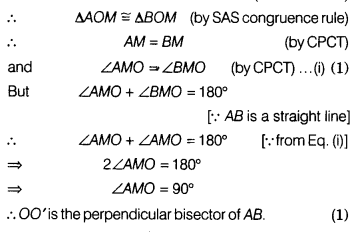# It two circles intersect in two points, prove that the line through their centres is the perpendiculae

It two circles intersect in two points, prove that the line through their centres is the perpendicular bisector of the common chord.

Let C(O, r) and O(O’, s) be two circles intersecting at points A and B.